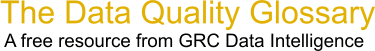Choose Index below for a list of all words and phrases defined in this glossary.

# First Time Yield (FTY)

index | Index

## First Time Yield (FTY) - definition(s)

First Time Yield - FTY - First Time Yield (FTY) is simply the number of good units produced divided by the number of total units going into the process. For example:

You have a process of that is divided into four sub-processes - A, B, C and D. Assume that you have 100 units entering process A. To calculate FTY you would:

1. Calculate the yield (number out of step/number into step) of each step. 2. Multiply these together.

For Example:

```   100 units enter A and 90 leave. The FTY for process A is 90/100 = .9
90 units go into B and 80 units leave. The FTY for process B is 80/90 = .89
80 units go into C and 75 leave. The FTY for C is 75/80 = .94
75 units got into D and 70 leave. The FTY for D is 70/75 = .93
```

The total process yield is equal to FTYofA * FTYofB * FTYofC * FTYofD or .9.89.94*.93 = .70.

You can also get the total process yield for the entire process by simply dividing the number of good units produced by the number going in to the start of the process. In this case, 70/100 = .70 or 70 percent yield.

First Time Yield Or First "Pass" Yield Is A Tool For Mearsuring The Amount Of Rework In A Given Process. It Is An Excellent Cost Of Quality Metric.

[Category=Data Quality ]

Source: iSixSigma, 22 January 2011 08:40:34, http://www.isixsigma.com/index.php?option=com_glossaryData Quality Glossary.  A free resource from GRC Data Intelligence. For comments, questions or feedback: dqglossary@grcdi.nl